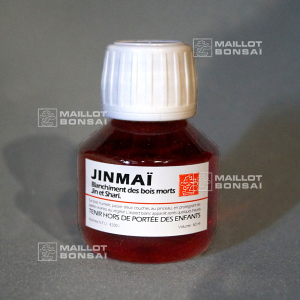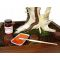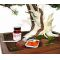##### The Japanese Bonsai specialist
Direct order Contact Help / Services Newsletter# Jin liquid

Yokozairef. : 4450

6,10

Available quantity : 92Order

###### Description

Size 50 ml. Plastic bottle. Protect the deadwood of jin and shari with this solution. This treatment is supposed to be used in winter when deciduous plants are dormant and without buds or leaves. Wash the trunk to remove moss, lichens and snails at the end of November or the beginning of December, and again at the end of January or early February. Use jin liquid on wet wood and apply two coats with a brush, whilst protecting the living parts of the bonsai. After a few hours you’ll see a white colour appear. Take care to protect the earth and pot with newspaper when applying this liquid. Use outside and keep out of reach of children.

For deciduous trees use one part of the product for 10 product.

For conifers use one part of the product to 30 parts of water.

#liquid 4.6 #product 3.7 #shari 3.5 #with 3.4 #this 3.4 #deciduous 2.9 #protect 2.7 #parts 2.5 #tools 2.5 #part 2.4

Formule
(( ROUND((CHAR_LENGTH(b.article_nom)-CHAR_LENGTH(REPLACE(b.article_nom, 'liquid', '')))/LENGTH('liquid')) + ROUND((CHAR_LENGTH(b.article_description)-CHAR_LENGTH(REPLACE(b.article_description, 'liquid', '')))/LENGTH('liquid')) ) * 4.6) + (( ROUND((CHAR_LENGTH(b.article_nom)-CHAR_LENGTH(REPLACE(b.article_nom, 'product', '')))/LENGTH('product')) + ROUND((CHAR_LENGTH(b.article_description)-CHAR_LENGTH(REPLACE(b.article_description, 'product', '')))/LENGTH('product')) ) * 3.7) + (( ROUND((CHAR_LENGTH(b.article_nom)-CHAR_LENGTH(REPLACE(b.article_nom, 'with', '')))/LENGTH('with')) + ROUND((CHAR_LENGTH(b.article_description)-CHAR_LENGTH(REPLACE(b.article_description, 'with', '')))/LENGTH('with')) ) * 3.4) + (( ROUND((CHAR_LENGTH(b.article_nom)-CHAR_LENGTH(REPLACE(b.article_nom, 'this', '')))/LENGTH('this')) + ROUND((CHAR_LENGTH(b.article_description)-CHAR_LENGTH(REPLACE(b.article_description, 'this', '')))/LENGTH('this')) ) * 3.4) + (( ROUND((CHAR_LENGTH(b.article_nom)-CHAR_LENGTH(REPLACE(b.article_nom, 'deciduous', '')))/LENGTH('deciduous')) + ROUND((CHAR_LENGTH(b.article_description)-CHAR_LENGTH(REPLACE(b.article_description, 'deciduous', '')))/LENGTH('deciduous')) ) * 2.9) + (( ROUND((CHAR_LENGTH(b.article_nom)-CHAR_LENGTH(REPLACE(b.article_nom, 'protect', '')))/LENGTH('protect')) + ROUND((CHAR_LENGTH(b.article_description)-CHAR_LENGTH(REPLACE(b.article_description, 'protect', '')))/LENGTH('protect')) ) * 2.7) + (( ROUND((CHAR_LENGTH(b.article_nom)-CHAR_LENGTH(REPLACE(b.article_nom, 'parts', '')))/LENGTH('parts')) + ROUND((CHAR_LENGTH(b.article_description)-CHAR_LENGTH(REPLACE(b.article_description, 'parts', '')))/LENGTH('parts')) ) * 2.5) + (( ROUND((CHAR_LENGTH(b.article_nom)-CHAR_LENGTH(REPLACE(b.article_nom, 'part', '')))/LENGTH('part')) + ROUND((CHAR_LENGTH(b.article_description)-CHAR_LENGTH(REPLACE(b.article_description, 'part', '')))/LENGTH('part')) ) * 2.4) + (( ROUND((CHAR_LENGTH(b.article_nom)-CHAR_LENGTH(REPLACE(b.article_nom, 'when', '')))/LENGTH('when')) + ROUND((CHAR_LENGTH(b.article_description)-CHAR_LENGTH(REPLACE(b.article_description, 'when', '')))/LENGTH('when')) ) * 2.4) + (( ROUND((CHAR_LENGTH(b.article_nom)-CHAR_LENGTH(REPLACE(b.article_nom, 'protecting', '')))/LENGTH('protecting')) + ROUND((CHAR_LENGTH(b.article_description)-CHAR_LENGTH(REPLACE(b.article_description, 'protecting', '')))/LENGTH('protecting')) ) * 2)

## Secure payment## Delivery

Our logistic partners :04 74 55 23 48
Pépinière MAILLOT-BONSAÏ
Le Bois Frazy
01990 RELEVANT - FRANCE
on appointmentThe Japanese Bonsai specialist
If you like Maillot Bonsaï, share on  facebook

Pensez à venir nous rendre visite sur rendez vous. 04 74 55 23 48 merci.

L'équipe Maillot bonsaï vous souhaite ses meilleurs vœux de bonheur, de santé et de réussite pour cette nouvelle année. C'est l'occasion pour nous de vous dire un IMMENSE MERCI d'avoir été à nos côtés cette année.

2023, c'est 365 jours où nous serons présents pour vous aider, vous guider et répondre à vos questions. Plus que jamais, nous continuerons à vous accompagner et à vous proposer des produits de qualité.   Cette nouvelle année sera riche en nouveautés, alors restez connecté pour ne rien rater !

Guy Maillot

OK